# Material Dispersion

Excerpt from Infrared Fibers and Their Applications

All dielectric materials are dispersive. This means that the refractive index varies with wavelength, i.e., n = f(λ). This may be seen in the typical dispersion curve for a dielectric material (NaCl) shown in Fig. 2.8. There are several ways to measure dispersion in transparent materials. A simple measure is the Abbe number, VD. The Abbe number is obtained by measuring the index at several key wavelengths, or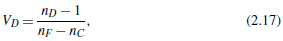where nF, nD, and nC, are the refractive indices at three standard visible wavelengths: λF = 486.1 nm (blue), λD = 589.2 nm (yellow), and λC = 656.3 nm (red). Abbe numbers are normally measured and tabulated for optical glasses that are transparent in the visible region. The most dispersive glasses are the heavier flint glasses with VD ranging from 30 to 40. Less dispersive glasses like the crown glasses have higher Abbe numbers. Silica has a VD = 68. Fluoride glasses have quite low dispersion, with VD ranging from 70 to 80.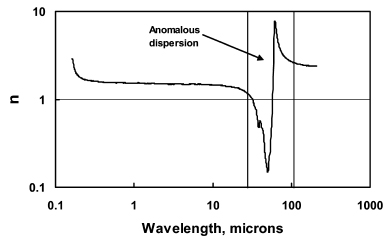Figure 2.8 Normal and anomalous dispersion for single-crystal NaCl over the visible and infrared spectral regions. [Reprinted from Ref. 1, Copyright 1985, with permission from Elsevier].

Another measure of dispersion is the derivative dn/dλ. The derivative may be most easily obtained from dispersion data like those shown in Fig. 2.8 once a curve fit to the data has been made to determine n as a function of wavelength. It is quite common to fit the dispersion data using a Sellmeier power-law fit. The Sellmeier equation is of the form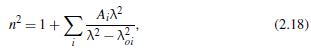where Ai and λoi are the Sellmeier constants: λoi is a vacuum wavelength related to natural vibrational frequencies λoiνoi = c. In general, a very good fit is obtained in the region of normal dispersion, with i = 3. The Sellmeier constants for many of the common IR materials are given in the Handbook of Optical Constants series by Palik.1

A pulse of light will travel with a group velocity vg. This pulse is a wavepacket composed of a spectrum of different frequencies (or wavelengths) centered at a frequency νo with a width ∆ν << νo. The group velocity is given by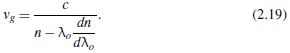The pulse itself broadens as a result of the individual components traveling with a different velocity. This leads to an overall temporal spreading of the pulse as it moves through the material. The temporal width στ (in ps) of a pulse after travelinga length L (in km) in a fiber is given by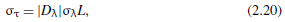where σλ is the initial source width (in nm); and Dλ is the dispersion coefficient for material dispersion (in ps/km-nm). Dλ is given by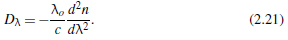The units for the dispersion coefficient are practical units more relevant to the long lengths of fibers used in telecommunication applications. In summary, it can be seen from Eqs. (2.19) and (2.21) that material dispersion affects pulse propagation though both the first derivative (group velocity) and the second derivative (dispersion coefficient).

As mentioned above, dn/dλ has a minimum value at the point of inflection in the dispersion curve. At the wavelength where dn/dλ is a minimum, d2n/dλ2 is zero and the group velocity is a maximum. This is the very important zero material dispersion wavelength (ZMD). In Fig. 2.12 data is shown for the dispersion coefficient Dλ for a typical fluoride glass (ZBLAN) and, for comparison, silica.2 The zero dispersion wavelengths occur at 1.724 μm and 1.312 μm for ZBLAN and silica, respectively. The zero dispersion wavelengths are always less than λmin, where the attenuation is least. For silica the minimum in attenuation occurs at 1.55 μm, and for ZBLAN at 2.45 μm. In modern telecommunication systems, both λmin and λZMD and the respective dispersion coefficients are very important in the design of both low-loss and low-dispersion systems.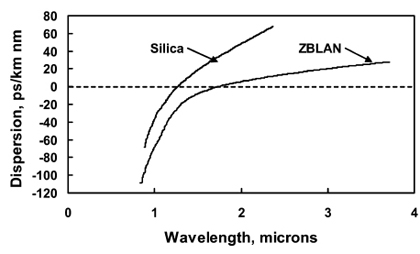Figure 2.12 Material dispersion, Dλ, for conventional silica fiber compared to that of the fluoride glass, ZBLAN. [Reprinted from Ref. 2, Copyright 1995, with permission from Elsevier].

### References

1. E. D. Palik, Handbook of Optical Constants of Solids, Academic Press, New York (1985).
2. F. Gan, "Optical properties of fluoride glasses: a review," J. Non-Cryst. Solids, Vol. 184, pp. 9-20 (1995).
Citation:

J. Harrington, Infrared Fibers and Their Applications, SPIE Press, Bellingham, WA (2003).